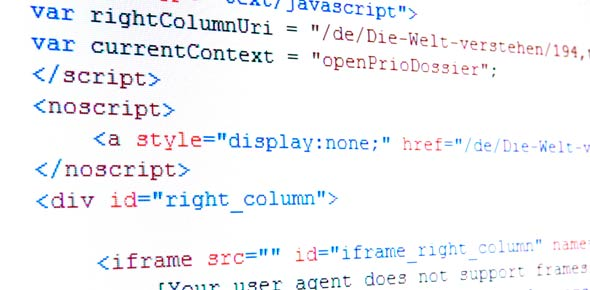# Quest Eduventuures Internship Test

60 Questions | Attempts: 134
ShareSettingsThis test is designed to check the analytical, logical and computer programming skills of an individual.

• 1.
Why is progamming important today?
• 2.
Why do you want to do internship?
• 3.
A memory location that holds a single letter or number.
• A.

Double

• B.

Int

• C.

Char

• D.

Word

• 4.
A do while and a while loop are the same
• A.

True

• B.

False

• 5.
A short sections of code written to complete a task.
• A.

Buffer

• B.

Array

• C.

Function

• 6.
Int hold decemals numbers
• A.

True

• B.

False

• 7.
What is FIFO?
• A.

First in Few Out

• B.

Few In Few out

• C.

First In First Out

• D.

Few In First Out

• 8.
What dose this equation mean ? a != t
• A.

A is assinged t

• B.

A and t are equal

• C.

A is not equal to t

• D.

• 9.
One loop inside the body of another loop is called
• A.

Loop in loop

• B.

Nested

• C.

Double loops

• 10.
Which data structure uses LIFO?
• A.

Array

• B.

Int

• C.

Stacks

• D.

Queues

• 11.
Entering Comments is a useless task, it will  not help in anyway.
• A.

True

• B.

False

• 12.
A Syntax Error is ?
• A.

An error you will never find

• B.

An error you find at the end when the program gives out a wrong value due to logic error

• C.

An error caused by language rules being broken.

• D.

An error due to user error

• 13.
What command do you use to output data to the screen?
• A.

Cin

• B.

Cout>>

• C.

Cout

• D.

Output>>

• 14.
Two main measures for the efficiency of an algorithm are
• A.

Processor and memory

• B.

Complexity and capacity

• C.

Time and space

• D.

Data and space

• 15.
The time factor when determining the efficiency of algorithm is measured by
• A.

Counting microseconds

• B.

Counting the number of key operations

• C.

Counting the number of statements

• D.

Counting the kilobytes of algorithm

• 16.
The space factor when determining the efficiency of algorithm is measured by
• A.

Counting the maximum memory needed by the algorithm

• B.

Counting the minimum memory needed by the algorithm

• C.

Counting the average memory needed by the algorithm

• D.

Counting the maximum disk space needed by the algorithm

• 17.
Which of the following case does not exist in complexity theory
• A.

Best case

• B.

Worst case

• C.

Average case

• D.

Null case

• 18.
The Worst case occur in linear search algorithm when
• A.

Item is somewhere in the middle of the array

• B.

Item is not in the array at all

• C.

Item is the last element in the array

• D.

Item is the last element in the array or is not there at all

• 19.
The Average case occur in linear search algorithm
• A.

When Item is somewhere in the middle of the array

• B.

When Item is not in the array at all

• C.

When Item is the last element in the array

• D.

When Item is the last element in the array or is not there at all

• 20.
The complexity of the average case of an algorithm is
• A.

Much more complicated to analyze than that of worst case

• B.

Much more simpler to analyze than that of worst case

• C.

Sometimes more complicated and some other times simpler than that of worst case

• D.

None or above

• 21.
The complexity of linear search algorithm is
• A.

O(n)

• B.

O(log n)

• C.

O(n2)

• D.

O(n log n)

• 22.
The complexity of Binary search algorithm is
• A.

O(n)

• B.

O(log )

• C.

O(n2)

• D.

O(n log n)

• 23.
The complexity of Bubble sort algorithm is
• A.

O(n)

• B.

O(log n)

• C.

O(n2)

• D.

O(n log n)

• 24.
The complexity of merge sort algorithm is
• A.

O(n)

• B.

O(log n)

• C.

O(n2)

• D.

O(n log n)

• 25.
The indirect change of the values of a variable in one module by another module is called
• A.

Internal change

• B.

Inter-module change

• C.

Side effect

• D.

Side-module update

## Related TopicsBack to top
×

Wait!
Here's an interesting quiz for you.